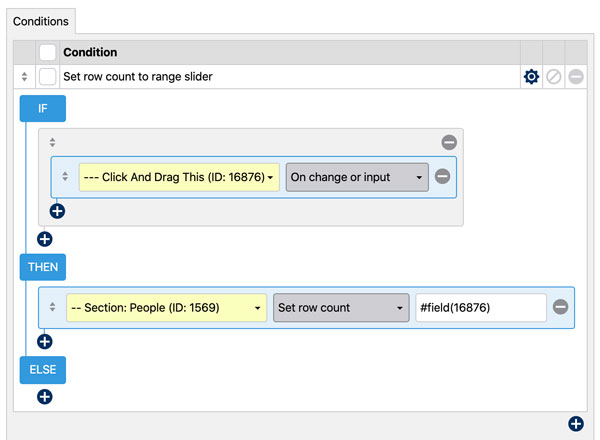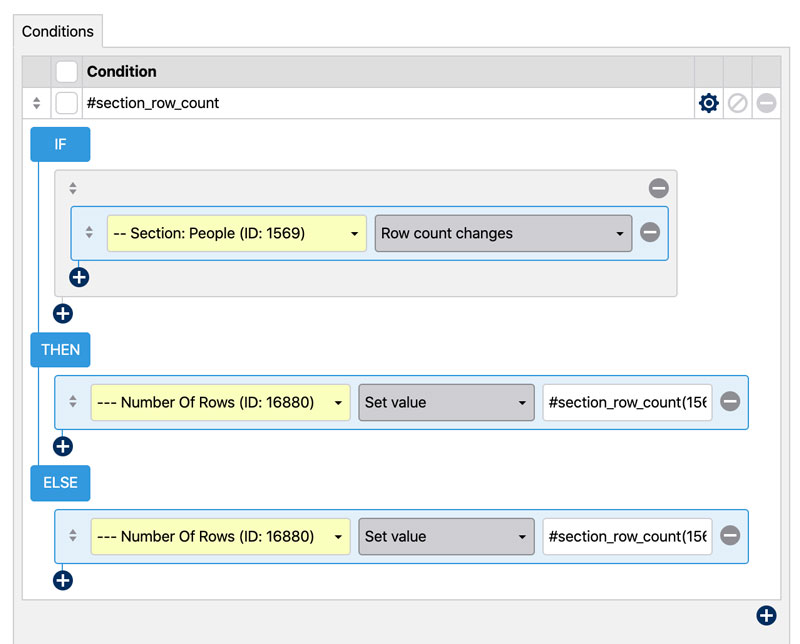# Use Fields To Change Repeatable Section Row CountsPRO

WS Form can set the number of rows in a repeatable section by using the value from another field. In the example below we are using the numeric value of a range slider to set the number of rows in the repeatable section. Instead of a range slider, this could be any other field that can accommodate a numeric value.

### Conditional Logic: Set Row Count

The demo above is achieved by using conditional logic. When selecting a section in a THEN or ELSE statement, you can select Set row count as an option. Note that this will only work with the section is set up as repeatable. We are using `#field(field_id)` to get the value of the range slider and using that to set the row count of the repeatable section People.

The conditional logic used in this demo to change the row count to match the range slider value is as follows:### Variable: #section_row_count(section_id)

You can also use the variable `#section_row_count(section_id)` to obtain the current number of rows in a repeatable section. You would set `section_id` to be the ID of the section you want to get row count from.

The conditional logic used in the demo to update the Number of Rows field is as follows:The ELSE statement above ensures that the Number of Rows field is initially updated with the row count before any interaction occurs. The THEN statement then updates the value after the People row count changes.